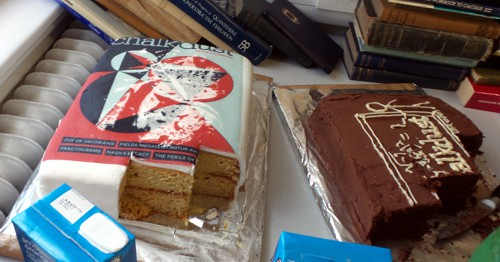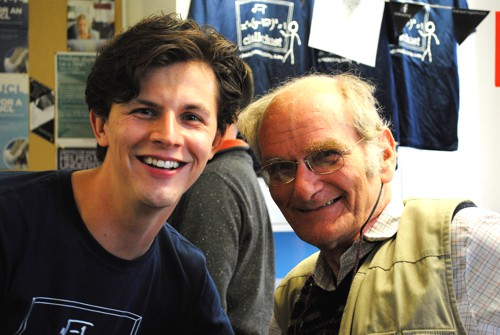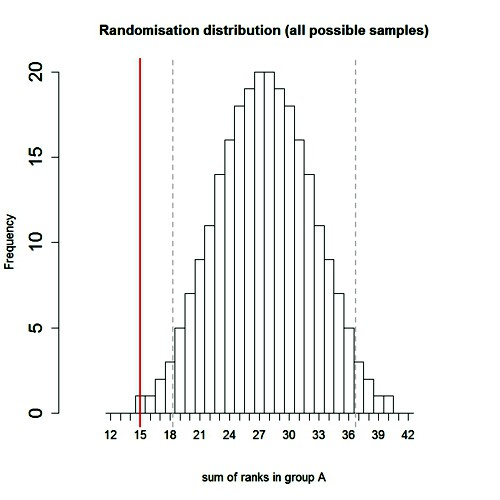Latest Tweets

Chalkdust is a magazine published by students of maths from UCL Mathematics department. Judging by its first issue, it’s an excellent vehicle for popularisation of maths. I have a piece in the second issue

You can view the whole second issue on line, or download a pdf of the whole issue. Or a pdf of my bit only: On the Perils of P values.

The piece started out as another exposition of the interpretation of P values, but the whole of the first part turned into an explanation of the principles of randomisation tests. It beats me why anybody still does a Student’s t test. The idea of randomisation tests is very old. They are as powerful as t tests when the assumptions of the latter are fulfilled but a lot better when the assumptions are wrong (in the jargon, they are uniformly-most-powerful tests).

Not only that, but you need no mathematics to do a randomisation test, whereas you need a good deal of mathematics to follow Student’s 1908 paper. And the randomisation test makes transparently clear that random allocation of treatments is a basic and essential assumption that’s necessary for the the validity of any test of statistical significance.

I made a short video that explains the principles behind the randomisation tests, to go with the printed article (a bit of animation always helps).

When I first came across the principals of randomisation tests, i was entranced by the simplicity of the idea. Chapters 6 – 9 of my old textbook were written to popularise them. You can find much more detail there.

In fact it’s only towards the end that I reiterate the idea that P values don’t answer the question that experimenters want to ask, namely:- if I claim I have made a discovery because P is small, what’s the chance that I’ll be wrong?

If you want the full story on that, read my paper. The story it tells is not very original, but it still isn’t known to most experimenters (because most statisticians still don’t teach it on elementary courses). The paper must have struck a chord because it’s had over 80,000 full text views and more than 10,000 pdf downloads. It reached an altmetric score of 975 (since when it has been mysteriously declining). That’s gratifying, but it is also a condemnation of the use of metrics. The paper is not original and it’s quite simple, yet it’s had far more "impact" than anything to do with my real work.

If you want simpler versions than the full paper, try this blog (part 1 and part 2), or the Youtube video about misinterpretation of P values.

The R code for doing 2-sample randomisation tests

You can download a pdf file that describes the two R scripts. There are two different R programs.

One re-samples randomly a specified number of times (the default is 100,000 times, but you can do any number). Download two_sample_rantest.R

The other uses every possible sample -in the case of the two samples of 10 observations,it gives the distribution for all 184,756 ways of selecting 10 observations from 20. Download 2-sample-rantest-exact.R

The launch party

Today the people who organise Chalkdust magazine held a party in the mathematics department at UCL. The editorial director is a graduate student in maths, Rafael Prieto Curiel. He was, at one time in the Mexican police force (he said he’d suffered more crime in London than in Mexico City). He, and the rest of the team, are deeply impressive. They’ve done a terrific job. Support them.The party cakesRafael Prieto doing the introductionRafael Prieto doing the introductionRafael Prieto and meI got the T shirt

Decoding the T shirt

The top line is "I" because that’s the usual symbol for the square root of -1.

 The second line is one of many equations that describe a heart shape. It can be plotted by calculating a matrix of values of the left hand side for a range of values of x and y. Then plot the contour for a values x and y for which the left hand side is equal to 1. Download R script for this. (Method suggested by Rafael Prieto Curiel.)### Follow-up

5 November 2015

The Mann-Whitney test

I was stimulated to write this follow-up because yesterday I was asked by a friend to comment on the fact that five different tests all gave identical P values, P = 0.0079. The paper in question was in Science magazine (see Fig. 1), so it wouldn’t surprise me if the statistics were done badly, but in this case there is an innocent explanation.

The Chalkdust article, and the video, are about randomisation tests done using the original observed numbers, so look at them before reading on. There is a more detailed explanation in Chapter 9 of Lectures on Biostatistics. Before it became feasible to do this sort of test, there was a simpler, and less efficient, version in which the observations were ranked in ascending order, and the observed values were replaced by their ranks. This was known as the Mann Whitney test. It had the virtue that because all the ‘observations’ were now integers, the number of possible results of resampling was limited so it was possible to construct tables to allow one to get a rough P value. Of course, replacing observations by their ranks throws away some information, and now that we have computers there is no need to use a Mann-Whitney test ever. But that’s what was used in this paper.

In the paper (Fig 1) comparisons are made between two groups (assumed to be independent) with 5 observations in each group. The 10 observations are just the ranks, 1, 2, 3, 4, 5, 6, 7, 8, 9, 10.

To do the randomisation test we select 5 of these numbers at random for sample A, and the other 5 are sample B. (Of course this supposes that the treatments were applied randomly in the real experiment, which is unlikely to be true.) In fact there are only 10!/(5!.5!) = 252 possible ways to select a sample of 5 from 10, so it’s easy to list all of them. In the case where there is no overlap between the groups, one group will contain the smallest observations (ranks 1, 2, 3, 4, 5, and the other group will contain the highest observations, ranks 6, 7, 8, 9, 10.

In this case, the sum of the ‘observations’ in group A is 15, and the sum for group B is 40.These add to the sum of the first 10 integers, 10.(10+1)/2 = 55. The mean (which corresponds to a difference between means of zero) is 55/2 = 27.5.

There are two ways of getting an allocation as extreme as this (first group low, as above, or second group low, the other tail of the distribution). The two tailed P value is therefore 2/252 = 0.0079. This will be the result whenever the two groups don’t overlap, regardless of the numerical values of the observations. It’s the smallest P value the test can produce with 5 observations in each group.

The whole randomisation distribution looks like thisIn this case, the abscissa is the sum of the ranks in sample A, rather than the difference between means for the two groups (the latter is easily calculated from the former). The red line shows the observed value, 15. There is only one way to get a total of 15 for group A: it must contain the lowest 5 ranks (group A = 1, 2, 3, 4, 5). There is also only one way to get a total of 16 (group A = 1, 2, 3, 4, 6),and there are two ways of getting a total of 17 (group A = 1, 2, 3, 4, 7, or 1, 2, 3, 5, 6), But there are 20 different ways of getting a sum of 27 or 28 (which straddle the mean, 27.5). The printout (.txt file) from the R program that was used to generate the distribution is as follows.

 Randomisation test: exact calculation all possible samples INPUTS: exact calculation: all possible samples Total number of combinations = 252 number obs per sample = 5 sample A 1 2 3 4 5 sample B 6 7 8 9 10 OUTPUTS sum for sample A= 15 sum for sample B = 40 mean for sample A= 3 mean for sample B = 8 Observed difference between sums (A-B) -25 Observed difference between means (A-B) -5 SD for sample A) = 1.581139 SD for sample B) = 1.581139 mean and SD for randomisation dist = 27.5 4.796662 quantiles for ran dist (0.025, 0.975) 18.275 36.725 Area equal to orless than observed diff 0.003968254 Area equal to or greater than minus observed diff 0.003968254 Two-tailed P value 0.007936508 Result of t test P value (2 tail) 0.001052826 confidence interval 2.693996 7.306004

Some problems. Figure 1 alone shows 16 two-sample comparisons, but no correction for multiple comparisons seems to have been made. A crude Bonferroni correction would require replacement of a P = 0.05 threshold with P = 0.05/16 = 0.003. None of the 5 tests that gave P = 0.0079 reaches this level (of course the whole idea of a threshold level is absurd anyway).

Furthermore, even a single test that gave P = 0.0079 would be expected to have a false positive rate of around 10 percent

## 7 Responses to The perils of P values, in Chalkdust magazine

This site uses Akismet to reduce spam. Learn how your comment data is processed.